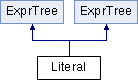# Literal Class Reference

`#include <literals.h>`

Inheritance diagram for Literal:List of all members.

## Public Member Functions

virtual ~Literal ()
Destructor.
Literal (const Literal &literal)
Copy constructor.
Literaloperator= (const Literal &literal)
Assignment operator.
virtual ExprTreeCopy () const
Make a deep copy.
void CopyFrom (const Literal &literal)
void GetComponents (Value &v, Value::NumberFactor &f) const
void GetValue (Value &v) const
virtual bool SameAs (const ExprTree *tree) const
virtual ~Literal ()
Destructor.
Literal (const Literal &literal)
Copy constructor.
Literaloperator= (const Literal &literal)
Assignment operator.
virtual ExprTreeCopy () const
Make a deep copy.
void CopyFrom (const Literal &literal)
void GetComponents (Value &v, Value::NumberFactor &f) const
void GetValue (Value &v) const
virtual bool SameAs (const ExprTree *tree) const

## Static Public Member Functions

static LiteralMakeAbsTime (abstime_t *now=NULL)
static LiteralMakeAbsTime (std::string timestr)
static LiteralMakeRelTime (time_t secs=-1)
static LiteralMakeRelTime (time_t t1, time_t t2)
static LiteralMakeRelTime (std::string str)
static LiteralMakeReal (std::string realstr)
static LiteralMakeLiteral (const Value &v, Value::NumberFactor f=Value::NO_FACTOR)
static int findOffset (time_t epochsecs)
static LiteralMakeAbsTime (abstime_t *now=NULL)
static LiteralMakeAbsTime (std::string timestr)
static LiteralMakeRelTime (time_t secs=-1)
static LiteralMakeRelTime (time_t t1, time_t t2)
static LiteralMakeRelTime (std::string str)
static LiteralMakeReal (std::string realstr)
static LiteralMakeLiteral (const Value &v, Value::NumberFactor f=Value::NO_FACTOR)
static int findOffset (time_t epochsecs)

Literal ()
Constructor.
Literal ()
Constructor.

## Friends

class FunctionCall
class ExprList
class Operation
bool operator== (Literal &literal1, Literal &literal2)
bool operator== (Literal &literal1, Literal &literal2)

## Detailed Description

Represents the literals of the ClassAd language, such as integers, reals, booleans, strings, undefined and real.

## Member Function Documentation

 void Literal::GetComponents ( Value & v, Value::NumberFactor & f ) const

Deconstructor to get the components of the literal

Parameters:
 v The encapsulated value f The number factor (invalid if v is non-numeric)
 void Literal::GetComponents ( Value & v, Value::NumberFactor & f ) const

Deconstructor to get the components of the literal

Parameters:
 v The encapsulated value f The number factor (invalid if v is non-numeric)
 void Literal::GetValue ( Value & v ) const

Deconstructor to get the encapsulated value

Parameters:
 v The value encapsulated by the literal
 void Literal::GetValue ( Value & v ) const

Deconstructor to get the encapsulated value

Parameters:
 v The value encapsulated by the literal
 Literal * Literal::MakeAbsTime ( abstime_t * now = `NULL` ) ` [static]`

Create an absolute time literal.

Parameters:
 now The time in UNIX epoch. If a value of NULL is passed in the system's current time will be used.
Returns:
The literal expression.
 static Literal* Literal::MakeAbsTime ( abstime_t * now = `NULL` ) ` [static]`

Create an absolute time literal.

Parameters:
 now The time in UNIX epoch. If a value of NULL is passed in the system's current time will be used.
Returns:
The literal expression.
 Literal * Literal::MakeLiteral ( const Value & v, Value::NumberFactor f = `Value::NO_FACTOR` ) ` [static]`

Factory method to construct a Literal

Parameters:
 v The value to convert to a literal. (Cannot be a classad or list value.) f The number factor (B, K, M, G, T) --- ignored for non-numeric values.
Returns:
The constructed literal expression
 static Literal* Literal::MakeLiteral ( const Value & v, Value::NumberFactor f = `Value::NO_FACTOR` ) ` [static]`

Factory method to construct a Literal

Parameters:
 v The value to convert to a literal. (Cannot be a classad or list value.) f The number factor (B, K, M, G, T) --- ignored for non-numeric values.
Returns:
The constructed literal expression
 static Literal* Literal::MakeRelTime ( time_t secs = `-1` ) ` [static]`

Create a relative time literal.

Parameters:
 secs The number of seconds. If a value of -1 is passed in the time since midnight (i.e., the daytime) is used.
Returns:
The literal expression.
 static Literal* Literal::MakeRelTime ( time_t t1, time_t t2 ) ` [static]`

Create a relative time interval by subtracting two absolute times.

Parameters:
 t1 The end time of the interval. If -1 is passed in, the system's current time will be used. t2 the start time of the interval If -1 is passed in, the system's current time will be used.
Returns:
The literal expression of the relative time (t1 - t2).
 Literal * Literal::MakeRelTime ( time_t secs = `-1` ) ` [static]`

Create a relative time literal.

Parameters:
 secs The number of seconds. If a value of -1 is passed in the time since midnight (i.e., the daytime) is used.
Returns:
The literal expression.
 Literal * Literal::MakeRelTime ( time_t t1, time_t t2 ) ` [static]`

Create a relative time interval by subtracting two absolute times.

Parameters:
 t1 The end time of the interval. If -1 is passed in, the system's current time will be used. t2 the start time of the interval If -1 is passed in, the system's current time will be used.
Returns:
The literal expression of the relative time (t1 - t2).
 bool Literal::SameAs ( const ExprTree * tree ) const` [virtual]`

Is this ExprTree the same as the tree?

Returns:
true if it is the same, false otherwise

Implements ExprTree.

 virtual bool Literal::SameAs ( const ExprTree * tree ) const` [virtual]`

Is this ExprTree the same as the tree?

Returns:
true if it is the same, false otherwise

Implements ExprTree.

The documentation for this class was generated from the following files: